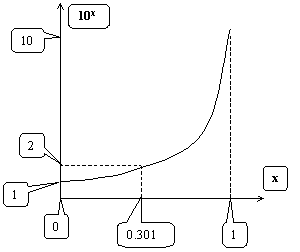EXPONENTIALS

ANIL MITRA PHD, COPYRIGHT © 2000, REFORMATTED June 25, 2003

EXPONENTIALS

1.    Rules

XA+B = XA . XB

XAB = (XA)B = (XB)A

Common values for the exponent X are e and 10. e, the base of “natural logarithms” is useful - especially in higher mathematics.

2.    Examples

 103 = 10x10x10 ex = lim n®0 [1+x/m]m 101 = 10 e = 2.71828 100 = 1 e0 = 1 X0 = 1 if X ¹ 0 10-3 = 1/103

Fractions

100.5 = 10(1/2) = z ® z2 = (101/2)2 = 10

or, 100.5 = 10(1/2) = 2Ö10

similarly

X0.6 =  10ÖX6

Any number

X7.6 = X7+0.6 = X7 . X0.6

3.    A graph